# FlightSimulator2

## FlightSimulator2

In this program, we will take a gigantic step forward in the quality of our game. This game will actually be a lot of fun to play, and quite challenging. If you have a computer with a processor slower than about 600 MHz, you will probably have trouble running this game and the ones that follow.

We wish to add several features to our game:

• Displays altitude, airspeed, rate of climb, pitch, and roll on screen

• Airspeed depends on pitch

• Aircraft climbs or drops with pitch

• Aircraft moves to right and left when rolling

• Aircraft yaws to right and left when rolling

• Allows the player to calculate vertical speed

• Determines if the landing was good based on location, heading, and vertical speed

### Equation for Speed as a Function of Pitch

As in real-life aircraft, the airspeed of our jet is going to depend on the pitch. Speed will be highest when the aircraft is pointed straight down, and lowest when the aircraft points straight up. Anything in-between will have some intermediate value.

We then need an equation that will express this; you may have guessed that it will involve the sine of the pitch angle. We will use thetax as the pitch angle. (Incidentally, theta is a Greek letter often used in mathematics to name angles; the x denotes that the measurement is about the x-axis.) We will make upward pitches positive and downward pitches negative. Level flight will have zero pitch. As pitch angle increases , the sine of the pitch angle will be increasing too, so we can say ( assuming 1000 is the speed in level flight)

` airspeed = 1000  1000*Sin(thetax); `

Notice what will happen to airspeed as the plane pitches up (see Figure 5.13). The angle thetax becomes positive and increases. The sine of thetax increases also, so airspeed goes down. If the plane pitches down, thetax becomes negative, and so does the sine of thetax , so airspeed then increases. But is this totally realistic? Probably not, but it produces realistic behavior. For example, the equation we are using for airspeed requires the jet s speed to be 0 when the aircraft is pointing straight up, and 2000 when pointing straight down, which may not necessarily be the case. In fact, the F-16 aircraft can climb vertically at a considerable speed! However, as mentioned earlier the general behavior of the model is good, in that speed increases when the nose goes down, and decreases when it points up. If we really wanted, we could write equations for the exact speed of the F-16 based on pitch, but to do so is beyond the scope of this book.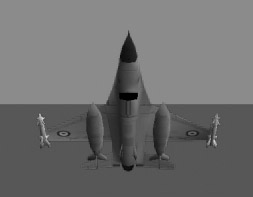Figure 5.13: Jet pitching up. Airspeed goes down.

Once we know the airspeed, we can move the aircraft according to that speed:

` // Move the aircraft as per the airspeed. speed = airspeed/100.; myjet.Move(speed); `

The last two lines bear some explaining. We have already seen the Move command: it moves the object in the direction in which it s pointing. When we do not specify the time in which the motion is to take place (as in Move(1500,2) , which would move the aircraft 1500 units in 2 seconds), the motion occurs immediately. In the code above, myjet is moved immediately a distance equal to speed .

### Computer Speed Issues

In the preceding code, you will also notice that the value of airspeed calculated is divided by 100 to come up with the value of speed , which goes in the Move statement.

Why was the airspeed value that we went through so much trouble to calculate divided by 100? The answer is that it was done to produce a realistic feeling when the program is actually run. Just because we picked somewhat realistic sounding numbers for the airspeed does not mean that they will result in realistic motion when applied to the Move command. The designers of Jamagic did not design the Move command to give realistic F-16 movement, nor should they have! It s our job to make the game realistic.

Dividing the airspeed by 100 resulted in acceptable motion with the particular computer used to make this game (a 900-MHz processor), but if you have a faster or slower computer, you will probably have to use a different number; you will have to experiment with this. It is possible to come up with algorithms to automatically adjust the value 100 to a more appropriate one based on the computer s speed. For example, at the very beginning of the game, you could have the computer move an object on the screen for a second or two, and then calculate the distance it moved. Based on this distance, you could adjust the value that is currently set to 100. For now, keep in mind that you may have to adjust this and other values to ensure proper action.

Why should the speed of the computer affect the speed of the motion? After all, it s supposed to happen immediately if the time element is left from the parentheses in the Move command. Isn t what is meant by immediate the same regardless of the computer s speed? The answer is that the Move command will be inside the game loop, cycling continuously. Each time the program reaches the Move command, the aircraft will move per the instructions, but because a faster computer will be looping faster, the aircraft will be moved the same distance each time, but more often. This difference can be enough to significantly affect how the game plays.

### Finding the Jet s Angle

You saw that we need to know the jet s pitch angle ( thetax , or angle the jet is making with its x-axis) in order to determine how fast the jet moves. We will also need to know the angle the jet is making with its y-axis (this is the yaw angle or thetay ), and the angle with the z-axis (the roll angle of the jet or thetaz ). We need to know the roll angle because the further the jet rolls to one side, the faster it will move toward that side as a result of the wings tilting to the side.

One of your first ideas might be to use the GetXAngle , GetYAngle , and GetZAngle functions to find these angles. However, there is a problem with this in that the values returned by these functions can become distorted when the jet rotates about all three axes ”at least as far as the jet is concerned . We need to then keep track of how much rotation is happening about each axis, and we can do so very easily. For example, we can keep track of the jet s roll by the following command, which is inserted in the code that rolls the jet to the right:

` If(Keyboard.IsKeyDown(Keyboard.RIGHT))  {    myjet.RollRight(Pi/500.);    thetaz = thetaz + (Pi/500.);    If(thetaz>(2*Pi))      {      thetaz = 0.0;         }  } `

You can figure it out easily: thetaz is the roll angle, and each time the code above is executed the jet is rolled pi/500 to the right. By adding pi/500 to thetaz we are keeping track of the angle of roll of the jet. When the jet rolls to the other side, we can subtract pi/500 from thetaz.

In the event that the aircraft rolls more than 360 degrees (2 pi) in one direction, we would like to reset the angle to 0 as soon as one revolution is complete. This explains the last lines of this code, which set thetaz to 0 as soon as one revolution was complete.

The same procedure can be followed for keeping track of the angle relative to the x-axis, as shown in the here:

` If(Keyboard.IsKeyDown(Keyboard.UP))  {    myjet.TurnUp(Pi/3000.);    thetax = thetax  (Pi/3000.);    If(thetax<(2*Pi))      {      thetax = 0.0;      }   } If(Keyboard.IsKeyDown(Keyboard.DOWN))  {    myjet.TurnDown(Pi/3000.);    thetax = thetax + (Pi/3000.);    If(thetax>(2*Pi))      {      thetax = 0.0;      }   } `

### Vertical Speed

Next , we would like to know the jet s speed in the vertical direction. For example, let s say that you were riding your bicycle down a mountain that is 1 mile high, and you get to the bottom in 1/2 hour. Your bike speedometer was reading 20 miles per hour . What was your vertical speed?

Even though you were moving on the roadway at 20 miles per hour, your vertical speed was 1 mile every 1/2 hour, or 2 miles per hour.

Another example of the vertical speed (or rate of climb, as it s know in aviation) can be seen by considering an aircraft at take-off. The jet is flying at 500 miles per hour airspeed (that is, the speed of the aircraft in the direction it s pointing, relative to the air around it) and it climbs to an altitude of 6 miles in one hour. The jet s rate of climb or vertical speed is 6 miles per hour, even though the aircraft has an airspeed of 500 miles per hour. Figure 5.14 illustrates what happens.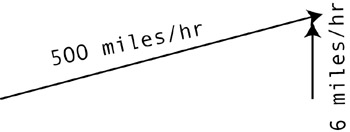Figure 5.14: An aircraft moves 500 miles in one hour and climbs 6 miles.

If we know the aircraft speed and pitch angle, we can easily find its vertical speed using the sine function. You will probably remember from the previous section that the sine of the angle is the spot where the horizontal projection of the angle hits the y-axis. But if instead of using a unit circle with a radius of one, we use a unit circle with a radius equal to the aircraft s airspeed (say 500), then the horizontal projection from the angle to the y-axis will hit the y-axis at a spot 500 times greater than before. This spot on the y-axis will give the value of the vertical speed. Bottom line: the airspeed times the sine of the angle of pitch gives the speed in the vertical direction. In Figure 5.15, for example, the jet moves at 500 miles per hour at an angle of 10 degrees. Its vertical speed is then

` 500 * sin (10) = 500 * .156 = 78 miles per hour. `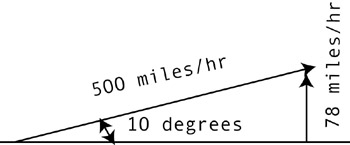Figure 5.15: The sine of the aircraft angle times its airspeed is the speed in the vertical direction (rate of climb).

Now that we have found the vertical speed of the aircraft using the sine function, then the value of the rate of climb vert is sent to a text box:

` vert = airspeed * sin(thetaz); mytext6.SetText("Vertical Speed: " + vert); `

We note that the angle thetaz must be in radians for the sine and cosine functions to work properly. If we wish to convert it to degrees we simply say

` degrees = thetaz * 360./(2.*Pi); `

### Yaw

You may remember that we need to make the aircraft move sideways and yaw when the aircraft rolls. We also want to make the jet move and yaw faster with increasing roll angles, which is consistent with real aircraft operation. We will use a variable called leftfactor to move the jet to the side, and a variable called addfactor to yaw it, combined with the cosine of the roll angle thetaz . The cosine of thetaz is 0 at 0 roll angle, and grows to a value of 1 when thetaz is 90 degrees:

` //Move the jet to the side when jet rolls. // Right roll first If(thetaz >0.01)     {      leftfactor = 0.0004 * Cos(thetaz);      myjet.MoveRight(leftfactor);      addfactor = Pi/1500. * Cos(thetaz);   myjet.AddAngle(0,addfactor,0);      thetay = thetay+addfactor;      If(thetay>(2*Pi))         {          thetay = 0.0;         }      } // // Roll to left. If(thetaz < 0.01)      {      leftfactor = 0.0004 * Cos(thetaz);      myjet.MoveLeft(leftfactor);      addfactor = Pi/1500. * Cos(thetaz);      myjet.AddAngle(0,addfactor,0);      thetay = thetay+addfactor;      If(thetay < (2*Pi))       {        thetay=0.0;       } `

You can see that the bigger thetaz is from 0 to 90 degrees, the larger the value of its cosine and the faster the motion of the jet when moving to the right. The statement myjet.AddAngle(0,addfactor,0) immediately rotates the jet about the y-axis (yaw) addfactor radians. If rotation had been required about the x- and z-axes, we could have used non-zero values in the parentheses. Of course, since we are only interested in yawing the aircraft, we only need to specify rotation about the one axis.

The numbers 0.004 and pi/500 (=3.14159/500) were arrived at by trial and error. With the computer used to make this game, these numbers gave satisfactory motion of the jet, however, you may need to adjust these numbers in your game.

### SetFocus Command

We want the camera to follow behind the jet instantaneously, so we use the Follow command. You may remember that the Optimize command is used in conjunction with SetStatic to ensure efficient use of the processor. The SetFocus line in the following code can be used to change how much of the screen is visible to the camera ”something like looking through the naked eye versus looking through a telescope . A value of 30 is about right for us; using a larger value would tend to widen the field of view, making objects smaller, and using a smaller value would decrease the field of view, making things larger and tending to distort the objects.

` // Camera ocamera1 = New Camera(oworld,owindow1); ocamera1.SetBackgroundColor(GetRGB(0,150,200)); ocamera1.Follow(myjet,100); oworld.Optimize(ocamera1); ocamera1.SetFocus(30); `

Figures 5.16 and 5.17 show the differences between focus values of 10 and 50.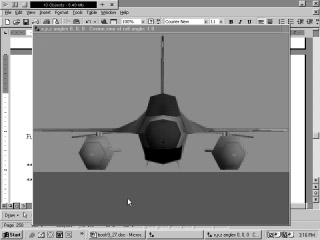Figure 5.16: The jet with a focus value of 10.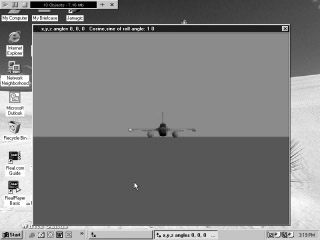Figure 5.17: The jet with a focus value of 50.

We now want to be able to switch back and forth from a camera angle directly behind the jet to one from the side and behind. The following code does this using the Shift and Caps Lock keys:

` //Set where to follow jet from. If(Keyboard.IsKeyDown(Keyboard.SHIFT))   { ocamera1.Follow(myjet,10,10,10,0);   } If(Keyboard.IsKeyDown(Keyboard.CAPSLOCK))   {     ocamera1.Follow(myjet,100);   } `

On the CD    The Jamagic CD-ROM comes with a very nice 3D image of an airport that is perfectly suited for our game. The airport can be found under Terrains in the CD-ROM of the full-capability version, and it can also be found under Terrains in the 3D menu of the book s Jamagic version.

The airport has a control tower, two runways, roads , fences, buildings , and a tarmac for waiting aircraft. The runways run east-west, and the jet shows up on the screen heading to the north. A proper landing can be made only from the east (aircraft heading west), a restriction that was put in to simulate real-world conditions in which aircraft must land in a direction into the wind. The pilot must then turn the aircraft around through 90 degrees to make a proper landing. Please see Figure 5.18, which shows the aircraft heading north, with the runways perpendicular .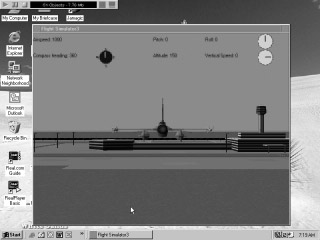Figure 5.18: The aircraft heads north. The runways run perpendicular east-west.

Here is the code that loads the airport, which we have elected to call mountain in order to avoid changing code as much as possible. It is scaled down considerably because of its size :

` // Airport oworld.Load("airport"); mountain = oworld.GetObject(oworld.GetNObjects()1); mountain.Scale(0.05,0.05,0.05); mountain.SetPosition(0,0,4000); mountain.SetStatic(); `

We want the pilot to know his heading by looking at the Heading Indicator on the screen, which is calculated fairly easily from the angle of the jet thetay about the y-axis. The angle thetay is in radians, and we convert it to degrees using the procedure of multiplying by 360 and dividing by 2 pi. In addition, we subtract the calculated value from 360 since a right turn from a 0 heading caused the heading to go to 359, where we need a right turn from zero heading to cause the degrees to increase:

` //Calculate compass heading in degrees. degs = 360.  thetay * 360.0/(2.*Pi) ; If (degs>360)  {    degs = degs  360;   } mytext2.SetText("Compass heading: " + degs); `

In addition, we would like the heading to be displayed as a compass direction, such as north or east. Please see Figure 5.19 to see the relationship between the compass directions and degree headings.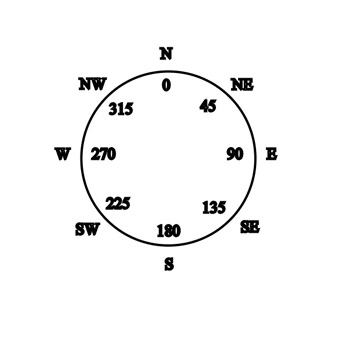Figure 5.19: Compass directions and corresponding degree headings.

The compass direction is determined using several if statements that match up a compass direction with a degree heading. In order to print the proper compass heading, we will be using a new type of variable called a string . You may recall that we have talked about floating point numbers, integers, and Booleans. Strings are one more type of variable, and they are used for text or letters . In this case, the variable ch will be a string, and it is initialized at the beginning of the program as ch = SOUTH . This lets Ja-magic know that ch is a string. The code below will make this clearer:

` If(degs > 22 && degs < 67)   {    ch = "NORTHEAST";    } If(degs > 67 && degs < 112)   {    ch = "EAST";    } If(degs > 112 && degs < 157)   {    ch = "SOUTHEAST";    } If(degs > 157 && degs < 202)   {    ch = "SOUTH";    } If(degs > 202 && degs < 247)   {    ch = "SOUTHWEST";    } If(degs > 247 && degs < 292)   {    ch = "WEST";    } If(degs > 292 && degs < 337)   {    ch = "NORTHWEST";    } If(degs > 337 && degs <= 360)   {    ch = "NORTH";    } If(degs >=0 && degs <= 22)   {    ch = "NORTH";    } // Print it to the screen. mytext7.SetText("COMPASS: " + ch); `

Notice that there are only eight directions. We could get a much finer resolution (NNE, SSW, and so on) using more if statements, but there is not much point to this since we will replace these written compass directions with a compass gauge that points in the proper direction in the next section.

### Criteria for a Successful Landing

The object of this game is to land the aircraft properly, which means several things:

• The vertical speed must not be too great upon landing (we chose 30 miles per hour as the maximum allowable speed ”it should probably be less than this, but the game becomes very difficult if the maximum allowable vertical speed is dropped much beyond this!)

• The airplane must have a heading between 268 degrees and 272 degrees (more or less parallel to the runways, in a westerly direction).

• The jet must land before the midpoint of the runway.

Care must be taken here in that the vertical speed when landing is negative (the plane is descending), so the criterion for allowable speed is that speed must be greater than “30.

Determining whether a proper landing happened occurs in the docollision function, called when there is any collision in the game. The aircraft touching down on the runway is a collision between the jet and the airport object. If the collision satisfies the above criteria, then the landing is deemed a successful one. If the above criteria are not met, a message informs the pilot that he has failed to land properly. The variables xco and zco are the x and z coordinates of the aircraft. Here is the code:

` Function docollision { // In order for landing to be successful several criteria // must be met: // Heading must be between 268 and 272 (must come in from the // east). // Vertical speed vert must be less than 30 miles per hour. // Landing must be between x = 2310 and 1770. // z must be between 6063 and 5241 (location of landing // area).       If(degs >= 268 && degs <=272)        {         If(vert>30 && zco 6063&& xco <1770 &&      xco> 2310)// one line      {         myjet.Stop();         SetText("Landed correctly!");         stopflag = TRUE;      RETURN stopflag;         }        Else          {              stopflag = TRUE;           myjet.Stop;              SetText("Bad landing!");           RETURN stopflag;          }       }  } `

stopflag is used to make sure that the jet stops, and the RETURN statement ensures that the value of stopflag ( TRUE or FALSE ) makes it back to the main program. The jet will stop if stopflag is TRUE since the command that moves the jet forward in the game loop has an if statement that requires stopflag to be false.

` If(stopflag == FALSE) {   myjet.Move(speed); } `

### More on Execution Speed

There are a few things to remember when you run the program: you may need to adjust the factors that move the jet to compensate for your computer s speed, and you may find that your computer is too slow if the processor is below about 600 MHz. In this case, you can try making your own landing surface using the CreatePlane command instead of using the airport (it has lots of polygons), much like we did with Sphere9 in Chapter 4. This should allow slower computers to use this code.

Another strategy you can use to try to improve the speed of execution is to change your computer color setting to as low a value as possible. This is done by going to your Windows Control Panel, selecting Display, and then clicking Settings. You will see a small window that allows you to change the colors to higher or lower values. A laptop computer with a 1.2-GHz processor was used to develop this section, and we noticed that the game was running no faster than it was on a 600-MHz desktop computer; in fact, the 600-MHz computer seemed faster! We switched the laptop s color setting from 32-bit to 16-bit, which considerably increased its speed, with no noticeable change in color quality. The desktop computer had been set to 16-bit color from the start.

You are encouraged to experiment with this game and make improvements. You may find that the aircraft moves too rapidly to the sides for your tastes ”the speed was selected partly to make the game easier to play and it is probably a little unrealistic in this sense. In addition, you can correct the bugs that occur if you bank the aircraft more than 90 degrees or climb at an angle greater than 90 degrees. Try it!

All in all, this is a very fun game to play, and it takes quite a bit of practice to land the jet properly.

### FlightSimulator2 Code

` // flightsimulator2 // Move aircraft in roll, pitch, and yaw // Import airport and check for proper landing. // Calculate heading, roll, pitch. // Use cosine function to move jet as it rolls in a // realistic fashion. // Calculate vertical speed using sine function. // Select between two views by clicking 'shift' or // 'caps lock' keys. // Use string variables for compass headings. oWindow1 = New Window("Flight Simulator2",640,480,Window.STANDARD);//one line //Window maintenance owindow1.SetAutoRefresh(OFF); // New world oworld = New World(); //Import jet. oworld.Load("f16"); myjet= oworld.GetObject(oworld.GetNObjects()1); myjet.Scale(0.01); myjet.SetPosition(0,150,2000); // Airport oworld.Load("airport"); mountain = oworld.GetObject(oworld.GetNObjects()1); mountain.Scale(0.05,0.05,0.05); mountain.SetPosition(0,0,4000); mountain.SetStatic(); // New cameras ocamera1 = New Camera(oworld,owindow1); ocamera1.SetBackgroundColor(GetRGB(0,150,200)); // Camera ocamera1.Follow(myjet,100); oworld.Optimize(ocamera1); ocamera1.SetFocus(30); // Text boxes mytext = New StaticText(owindow1," ",0,10,110,20); mytext.SetBackColor(GetRGB(0,150,200)); mytext.SetColor(GetRGB(0,0,0)); mytext2 = New StaticText(owindow1," ",0,40,150,20); mytext2.SetBackColor(GetRGB(0,150,200)); mytext2.SetColor(GetRGB(0,0,0)); mytext3 = New StaticText(owindow1," ",170,40,110,20); mytext3.SetBackColor(GetRGB(0,150,200)); mytext3.SetColor(GetRGB(0,0,0)); mytext4 = New StaticText(owindow1," ",170,10,140,20); mytext4.SetBackColor(GetRGB(0,150,200)); mytext4.SetColor(GetRGB(0,0,0)); mytext5 = New StaticText(owindow1," ",350,10,220,20); mytext5.SetBackColor(GetRGB(0,150,200)); mytext5.SetColor(GetRGB(0,0,0)); mytext6 = New StaticText(owindow1," ",350,40,220,20); mytext6.SetBackColor(GetRGB(0,150,200)); mytext6.SetColor(GetRGB(0,0,0)); mytext7 = New StaticText(owindow1," ",0,80,220,20); mytext7.SetBackColor(GetRGB(0,150,200)); mytext7.SetColor(GetRGB(0,0,0)); //Set collision detection. oworld.OnCollide = docollision; myjet.SetCollision(TRUE,Object.COLLISION_TYPE_STOP); // Initialize variables. airspeed = 0.0; alt = 0.0; thetax = 0.0; thetay = 0.0; thetaz = 0.0; degs = 0.0; speed = 0.0; addfactor = 0.0; leftfactor = 0.0; xpos = 0.; ypos = 150.; zpos = 0.0; Pitch2 = 0.0; roll2 = 0.0; rolldegs = 0.0; stopflag = FALSE; vert = 0.0; xco = 0.0; zco = 0.0; ch = "SOUTH"; //Game loop While(1) { // Window cleaning     ocamera1.ActivateRender();     owindow1.Refresh(); //Set where to follow jet from. If(Keyboard.IsKeyDown(Keyboard.SHIFT))   { ocamera1.Follow(myjet,10,10,10,0);   } // Jet controls If(Keyboard.IsKeyDown(Keyboard.CAPSLOCK))   {     ocamera1.Follow(myjet,100);   } If(Keyboard.IsKeyDown(Keyboard.LEFT))  {    myjet.RollLeft(Pi/500.);    thetaz = thetaz  (Pi/500.);    If(thetaz< (2*Pi))      {      thetaz = 0.0;      }  } If(Keyboard.IsKeyDown(Keyboard.RIGHT))  {    myjet.RollRight(Pi/500.);    thetaz = thetaz + (Pi/500.);    If(thetaz>(2*Pi))      {      thetaz = 0.0;  }  } If(Keyboard.IsKeyDown(Keyboard.UP))  {    myjet.TurnUp(Pi/3000.);    thetax = thetax  (Pi/3000.);    If(thetax<(2*Pi))      {      thetax = 0.0;      }   } If(Keyboard.IsKeyDown(Keyboard.DOWN))  {    myjet.TurnDown(Pi/3000.);    thetax = thetax + (Pi/3000.);    If(thetax>(2*Pi))      {      thetax = 0.0;      }   } //Calculate airspeed based on pitch. airspeed = 1000  1000*Sin(thetax); // Move the aircraft as per the airspeed. speed = airspeed/100.; If(stopflag == FALSE) {   myjet.Move(speed); } //Instruments mytext.SetText("Airspeed: "+ airspeed); //calculate compass heading in degrees degs = 360.  thetay * 360.0/(2.*Pi) ; If (degs>360)  {    degs = degs  360;   } mytext2.SetText("Compass heading: " + degs); //Altitude alt = myjet.GetY(); mytext3.SetText("Altitude: " + alt); // Convert to degrees. pitch = thetax * 360./(2.*Pi); mytext4.SetText("Pitch: " + Pitch);  //Calculate vertical speed. vert = airspeed * Sin(Pitch *2*Pi/360); mytext6.SetText("Vertical Speed: " + vert); //Convert radians to degrees. rolldegs = thetaz * 360./(2.*Pi); //Send the roll angle to the screen. mytext5.SetText("Roll: " + rolldegs); //Move the jet to the side when jet rolls. // Right roll first If(thetaz >0.01)      {      leftfactor = 0.0004 * Cos(thetaz);      myjet.MoveRight(leftfactor);      addfactor = Pi/1500. * Cos(thetaz);      myjet.AddAngle(0,addfactor,0);      thetay = thetay+addfactor;      If(thetay>(2*Pi))         {          thetay = 0.0;         }      } // // Roll to left. If(thetaz < 0.01)      {      leftfactor = 0.0004 * Cos(thetaz);      myjet.MoveLeft(leftfactor);      addfactor = Pi/1500. * Cos(thetaz);      myjet.AddAngle(0,addfactor,0);      thetay = thetay+addfactor;      If(thetay < (2*Pi))       {        thetay=0.0;       }      } // xco = myjet.GetX; zco = myjet.GetZ; yco = myjet.GetY; If(degs > 22 && degs < 67)   {    ch = "NORTHEAST";    } If(degs > 67 && degs < 112)   {    ch = "EAST";    } If(degs > 112 && degs < 157)   {    ch = "SOUTHEAST";    } If(degs > 157 && degs < 202)   {    ch = "SOUTH";    } If(degs > 202 && degs < 247)   {    ch = "SOUTHWEST";    } If(degs > 247 && degs < 292)   {    ch = "WEST";    } If(degs > 292 && degs < 337)   {    ch = "NORTHWEST";    } If(degs > 337 && degs <= 360)   {    ch = "NORTH";    } If(degs >=0 && degs <= 22)   {    ch = "NORTH";    } // Compass heading in north, south, etc. mytext7.SetText("COMPASS: " + ch); // End of game loop } Function docollision { // In order for landing to be successful several // criteria must be met: // Heading must be between 268 and 272 //(must come in from the east). // Vertical speed vert must be less than 30 miles per hour. // Landing must be between x = 2310 and 1770. // z must be between 6063 and 5241 (location of landing //area).       If(degs >= 268 && degs <=272)        {         If(vert>30 && zco 6063&& xco <1770 &&      xco> 2310)//one line         {         myjet.Stop();         SetText("Landed correctly!");         stopflag = TRUE;         Return stopflag;         }        Else         {          stopflag = TRUE;       myjet.Stop;          SetText("Bad landing!");          Return stopflag;         }        }  } `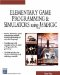Elementary Game Programming & Simulations Using Jamagic (Charles River Media Game Development)
ISBN: 1584502614
EAN: 2147483647
Year: 2002
Pages: 105
Authors: Sergio Perez